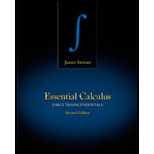# A vector equation for a line through the point ( 0 , 14 , − 10 ) and parallel to the line x = − 1 + 2 t , y = 6 − 3 t , z = 3 + 9 t and the parametric equations for a line through the point ( 0 , 14 , − 10 ) and parallel to the line x = − 1 + 2 t , y = 6 − 3 t , z = 3 + 9 t .### Essential Calculus: Early Transcen...

2nd Edition
James Stewart
Publisher: Cengage Learning
ISBN: 9781133112280### Essential Calculus: Early Transcen...

2nd Edition
James Stewart
Publisher: Cengage Learning
ISBN: 9781133112280

#### Solutions

Chapter 10.5, Problem 4E
To determine

## Expert Solution

### Want to see the full answer?

Check out a sample textbook solution.

### Want to see this answer and more?

Experts are waiting 24/7 to provide step-by-step solutions in as fast as 30 minutes!*

*Response times may vary by subject and question complexity. Median response time is 34 minutes for paid subscribers and may be longer for promotional offers.